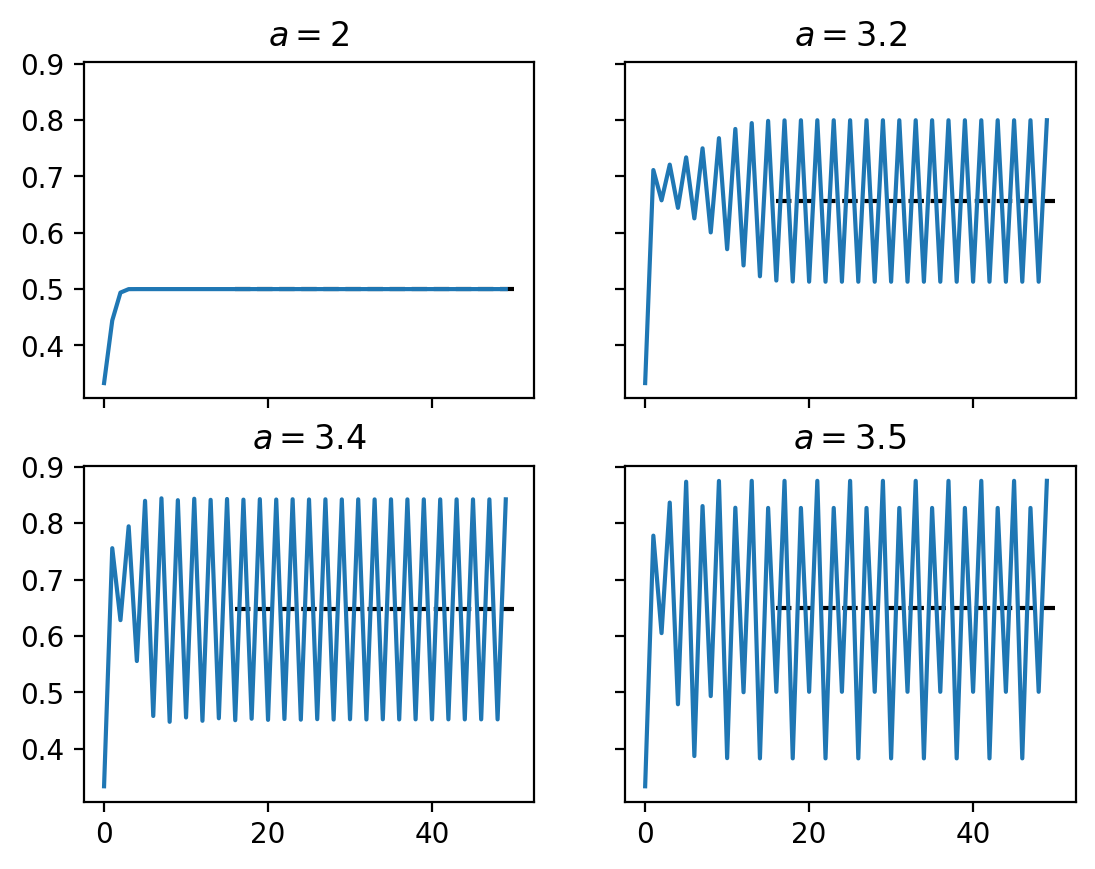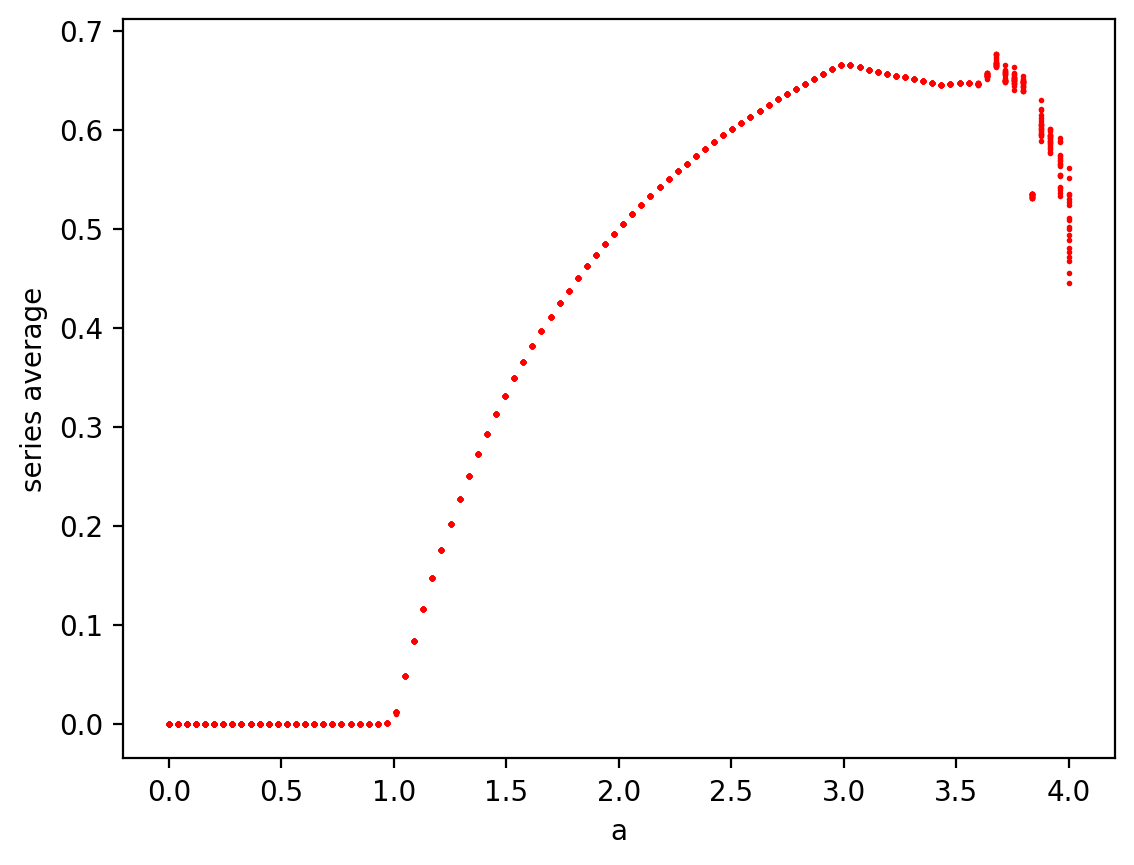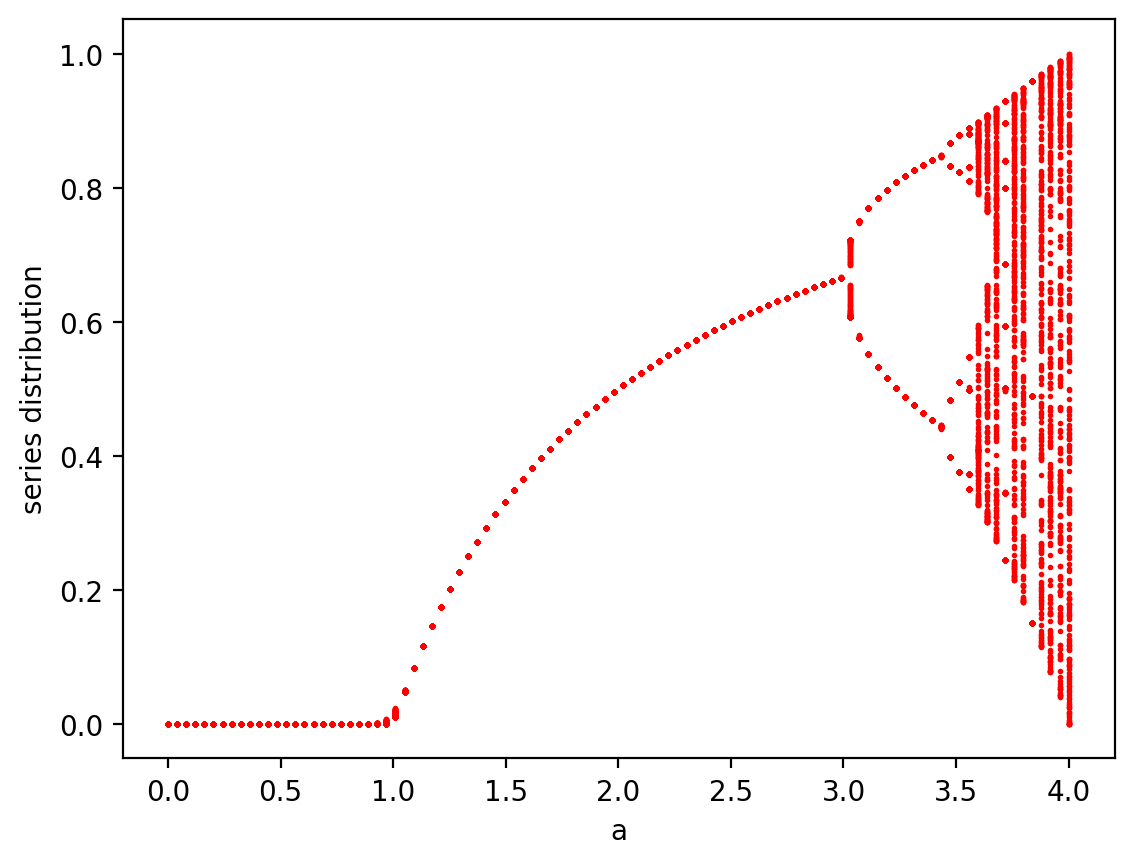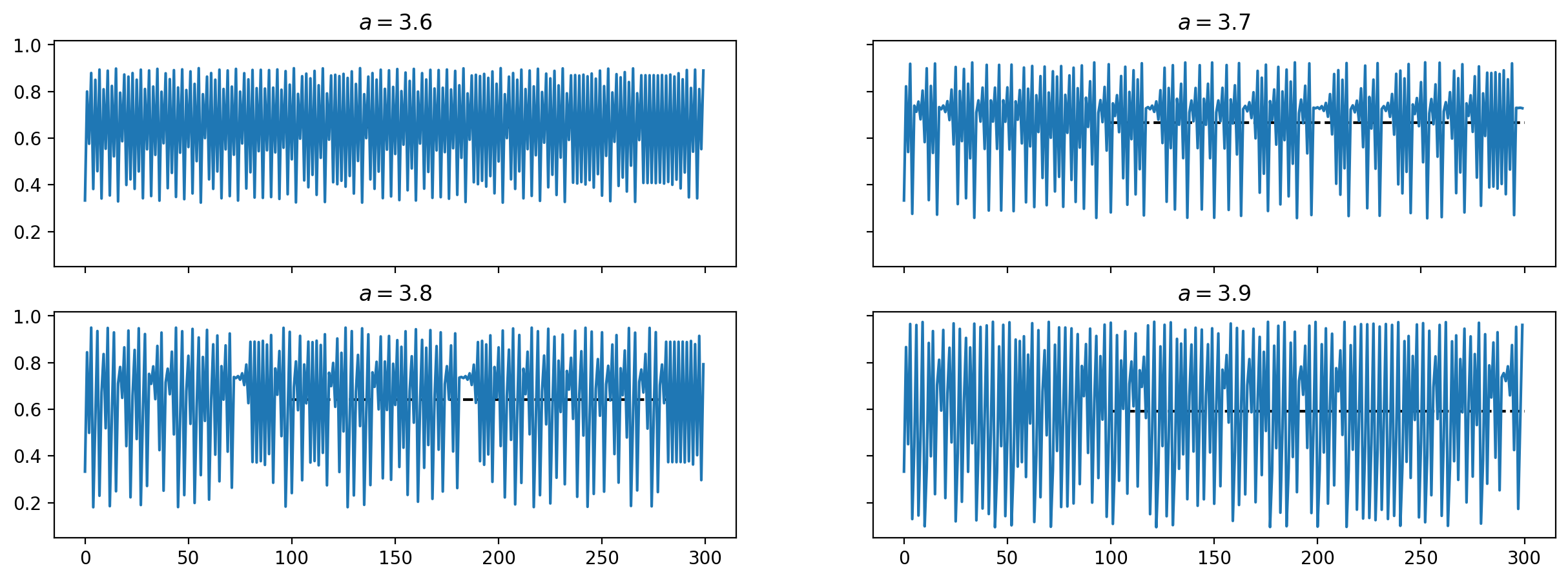# Jul 24 2019 Feigenbaum constant

Computing Feigenbaum constant using SymPy

So I saw this on Stephen Wolfram's blog where a simple recursive equation can yield chaotic behaviour and seems to have properties like Hopf bifurcation. I wanted to check out myself.

$$x_{i+1} = a x_i (1 - x_i)$$
In :
from sympy import *

## I would love to do it like this. Unfortunately I cannot find a nice way to make a recursive
## sequence in sympy. So I went for the pythonic approach. If someone has a better idea let me
## know!

# a, x = symbols('a x', real=True)
# i = symbols('i', integer=True)
# f, g = symbols('f g', cls=Function)
init_printing()

In :
from functools import lru_cache

@lru_cache(maxsize=8)
def f(i, a, x0):
if i == 0:
return x0
else:
return a * f(i-1, a, x0) * (1 - f(i-1, a, x0))

# sequence(f, (x, 0, 10))

In :
def series(n, a, x0=1/3): return [f(i, a, x0) for i in range(n)]
series(30, 3.2)

Out:
$\displaystyle \left[ 0.3333333333333333, \ 0.7111111111111111, \ 0.6573827160493827, \ 0.720738178204542, \ 0.6440789013854076, \ 0.7335720645618368, \ 0.6254210901002277, \ 0.7496625605058258, \ 0.6005395388213597, \ 0.7676537636274826, \ 0.5707566810113398, \ 0.7839791746952305, \ 0.5419386506861306, \ 0.7943716786516055, \ 0.5227050073850749, \ 0.7983503444468598, \ 0.5151586302990083, \ 0.7992646909678656, \ 0.5134100631677054, \ 0.7994245446586822, \ 0.5131038145790108, \ 0.7994505281391325, \ 0.5130540198310227, \ 0.799454696212004, \ 0.5130460317330444, \ 0.7994553633792654, \ 0.5130447530988549, \ 0.7994554701330878, \ 0.5130445485035884, \ 0.79945548721388\right]$
In :
from statistics import mean

def series_mean(n, a, x0=1/3):
s = series(n, a, x0)
return s, mean(s[n//3:])

In :
N = 50
fig, axes = plt.subplots(2, 2, sharex=True, sharey=True)
for a, ax in zip(
(2, 3.2, 3.4, 3.5),
axes.ravel()
):
s, smean = series_mean(N, a)
ax.plot(s)
ax.set_title(f'$a = {a}$')
ax.hlines(smean, N//3, N, linestyles='dashed')In the last subplot we begin to see period doublings.

# Bifurcation diagram¶

In the blog the following Wolfram code is used to generate the calculate the bifurcation. The first 50 values of the series are ignored to avoid transients and upto 300 values are calculated vfor each value of $a$.

ListPlot[Flatten[
Table[{a, #} & /@
Drop[NestList[Compile[x, a x (1 - x)], N[1/3], 300], 50], {a, 0,
4, .01}], 1], Frame -> True, FrameLabel -> {"a", "x"}]


Before we do that, let us see if it makes any difference if we vary the initial condition.

In :
import numpy as np
N = 300
a_values =  np.linspace(0, 4, 100)
x0_values = np.linspace(0.1, 5, 100)

plt.figure()
for x0 in x0_values:
smeans = [series_mean(N, a, x0) for a in a_values]
plt.scatter(a_values, smeans, s=1, c="r")
plt.xlabel("a")
plt.ylabel("series average")

/usr/lib/python3.7/site-packages/ipykernel_launcher.py:8: RuntimeWarning: overflow encountered in double_scalarsThe average value where the series oscillates around does not seem to depend on the value of $x_0$. So now instead of plotting the mean, we can plot the full distribution where of values where the series oscillates around.

In :
import numpy as np
N = 300
a_values =  np.linspace(0, 4, 100)

plt.figure()
for a in a_values:
s = series(N, a)[50:]
plt.scatter(a * np.ones_like(s), s, s=1, c="r")
plt.xlabel("a")
plt.ylabel("series distribution")There is our multiple Hopf bifurcation :) Let us see what happens for $a> 3.5$.

In :
N = 300
fig, axes = plt.subplots(2, 2, sharex=True, sharey=True, figsize=(15, 5))
for a, ax in zip(
(3.6, 3.7, 3.8, 3.9),
axes.ravel()
):
s, smean = series_mean(N, a)
ax.plot(s)
ax.set_title(f'$a = {a}$')
ax.hlines(smean, N//3, N, linestyles='dashed')Nice! Maybe I will do a follow up to compute the Lyapunov constant.

You can download this notebook, or see a static view on nbviewer.